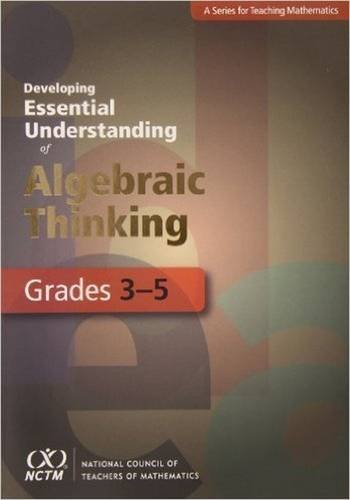# Developing Essential Understanding of Algebraic Thinking for Teaching Mathematics in Grades 3-5Type
Book
Authors

ISBN 10
0873536681
ISBN 13
9780873536684
Category
Books  [ Browse Items ]
Publication Year
2011
Pages
102
Abstract

Description
Like algebra at any level, early algebra is a way to explore, analyze, represent, and generalize mathematical ideas and relationships. This book shows that children can and do engage in generalizing about numbers and operations as their mathematical experiences expand. The authors identify and examine five big ideas and associated essential understandings for developing algebraic thinking in grades 3 5. The big ideas relate to the fundamental properties of number and operations, the use of the equals sign to represent equivalence, variables as efficient tools for representing mathematical ideas, quantitative reasoning as a way to understand mathematical relationships, and functional thinking to generalize relationships between covarying quantities. The book examines challenges in teaching, learning, and assessment and is interspersed with questions for teachers reflection. - from Amzon
Biblio Notes

Number of Copies